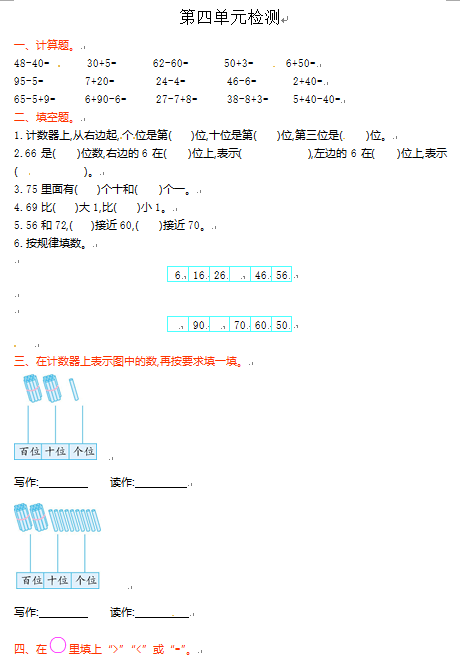48-40=　 　　30+5=　 　　62-60=　 　　50+3=　　　6+50=

95-5=            7+20=           24-4=         46-6=            2+40=

65-5+9=       6+90-6=           27-7+8=          38-8+3=        5+40-40=

1.计数器上,从右边起,个位是第(　　)位,十位是第(　　)位,第三位是(　　)位。

2.66是(　　)位数,右边的6在(　　)位上,表示(　　　　　　),左边的6在(　　)位上,表示(　　　　　　)。

【DOC文档4页】小学人教新课标数学一年级下学期第4单元测试卷1含答案 A4电子版资料_可直接打印_会员免费下载##### 评论信息